# vcjmhg 的个人博客

## 使用归并排序思想解决逆序对数量问题# 思路

## 拆分阶段## 合并阶段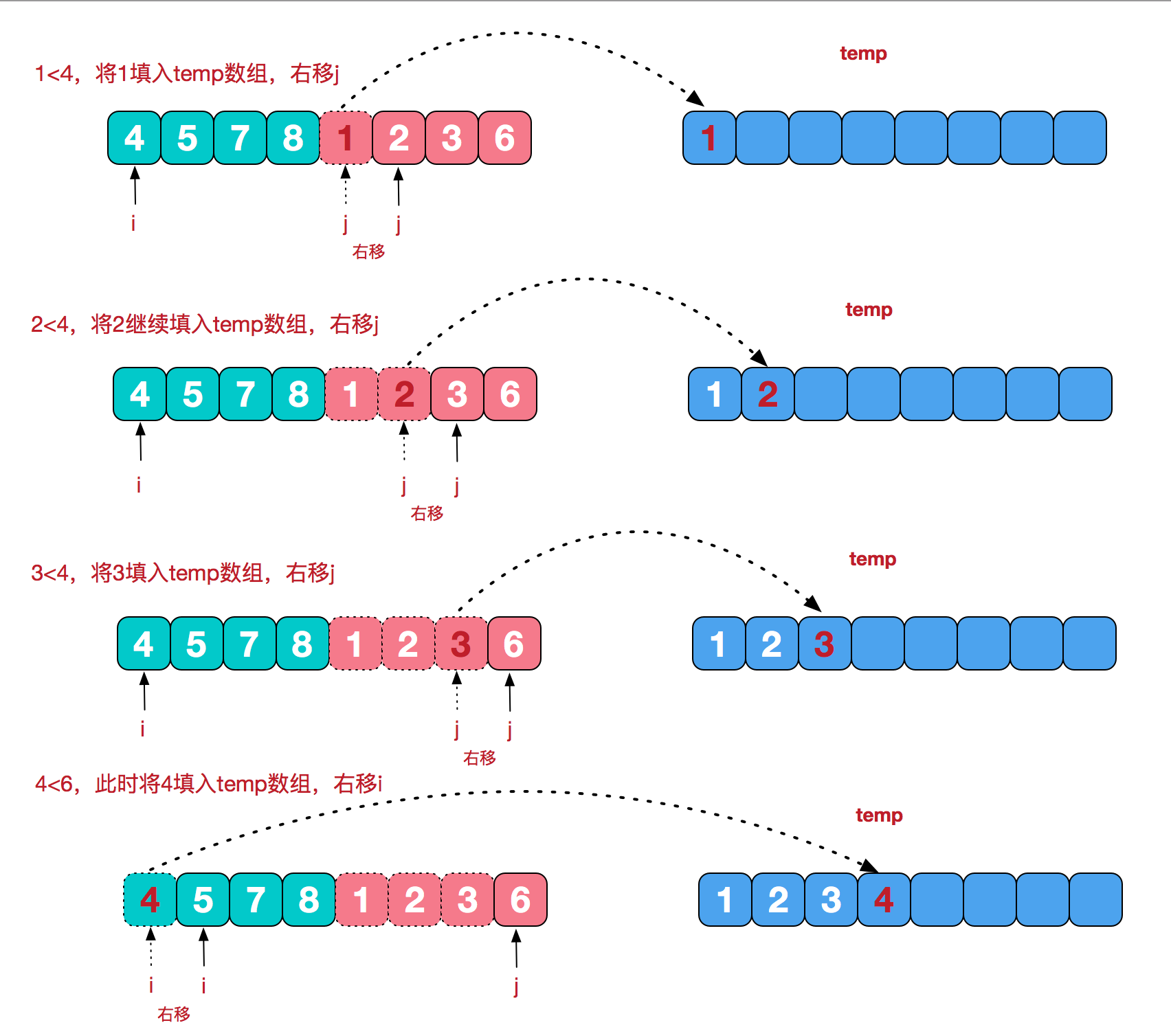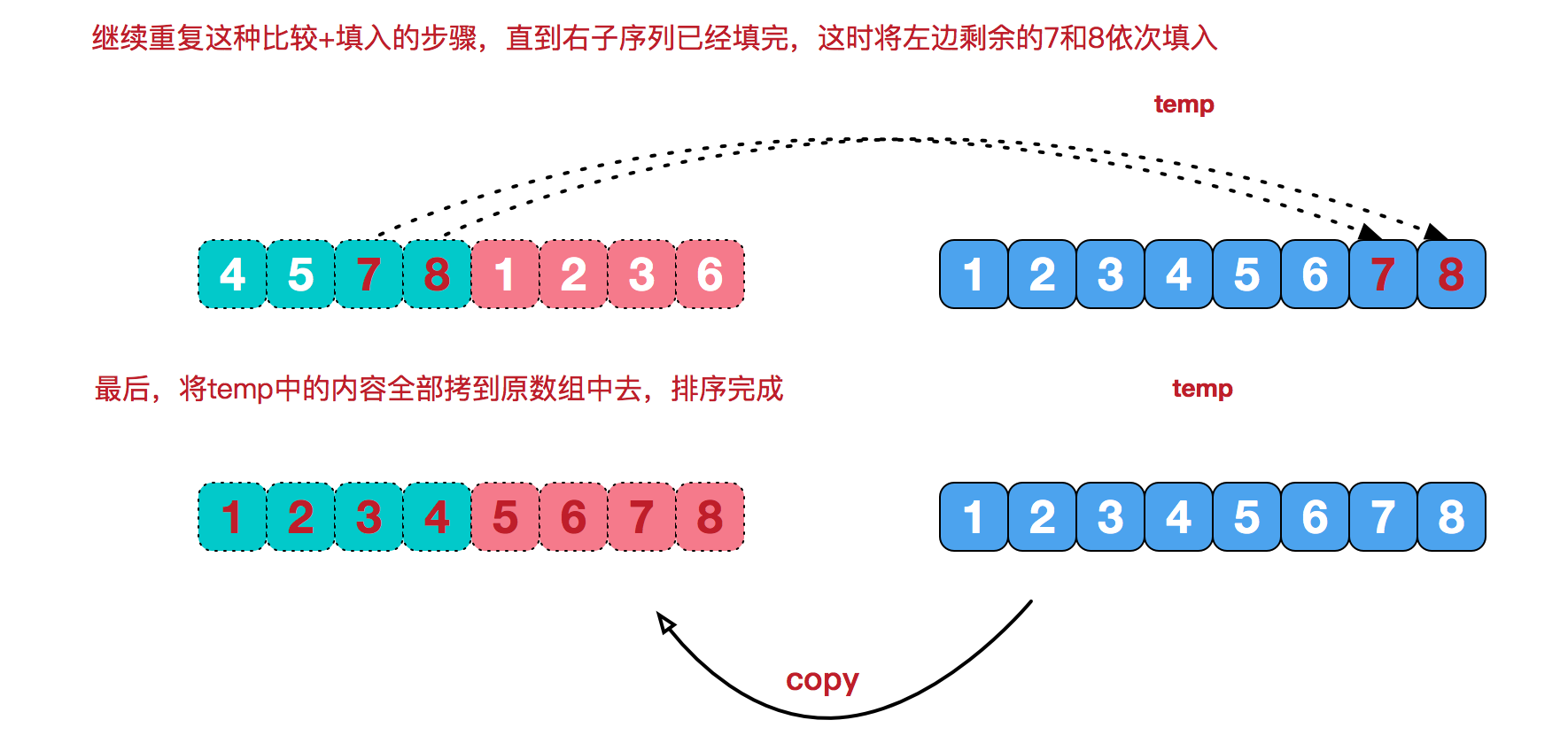# 实现

`````` 1	public static void mergeSort(int [] arr) {
2		int [] temp = new int [arr.length];
3		//通过辅助数组可以有效的利用空间，减少空间复杂度。防止sort时递归创建多个数组，增加空间开销
4		sort(arr, 0, arr.length - 1, temp);
5	}
6	/**对arr数组的[left...right]进行归并排序
7	**/
8	private static void sort(int [] arr, int left, int right, int [] temp) {
9		//保证排序边界的有效性
10		if(left < right) {
11			int mid = (right + left) / 2;
12			sort(arr, left, mid, temp);
13			sort(arr, mid + 1, right, temp);
14			merge(arr, left, mid, right, temp);
15		}
16	}
17	//将[left...mid]和[mid+1...right]进行合并
18	private static void merge(int [] arr, int left, int mid,int right, int [] temp) {
19		int i = left; //指向左侧索引
20		int j = mid +1; //指向右侧索引
21		int t = 0; //指向temp数组的指针
22		while(i <= mid && j <= right) {
23			if (arr[i] <= arr[j]) {
24				temp[t++] = arr[i++];
25			} else {
26				temp[t++] = arr[j++];
27			}
28		}
29		//未被合并的数组元素直接放到后面
30		while(i <= mid) temp[t++] = arr[i++];
31		while(j <= right) temp[t++] = arr[j++];
32		//将已经排序的temp数组中的元素复制到arr数组中
33		t = 0;
34		while(left <= right) arr[left++] = temp[t++];
35	}
``````

# 扩展与应用

## 剑指 Offer 51. 数组中的逆序对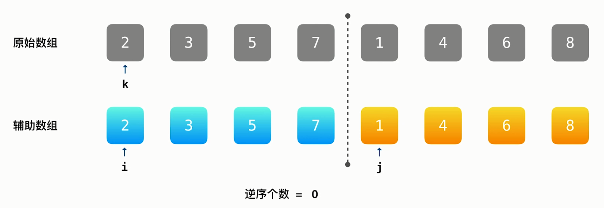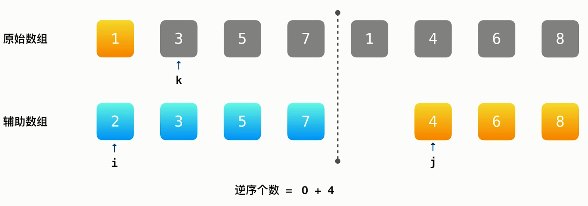i 后移，发现 3<4，直接将 3 放到第三个位置，同样未构成逆序对。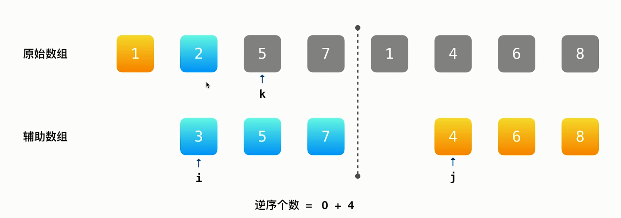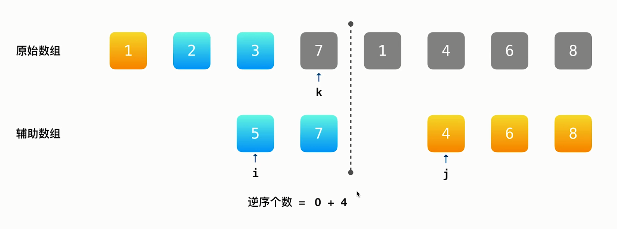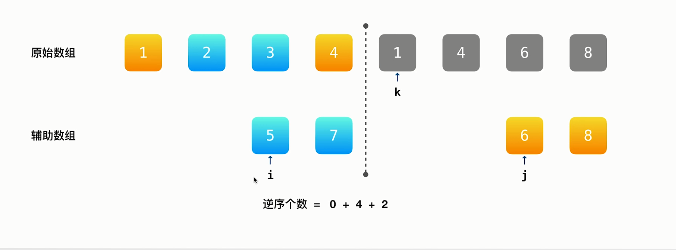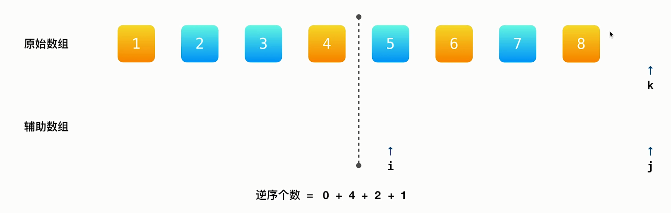## 代码实现

`````` 1/**
2使用归并排序思想来解决逆序对问题
3**/
4class Solution {
5    public int reversePairs(int[] nums) {
6        int [] temp = new int [nums.length];
7        return split(nums, 0, nums.length - 1, temp);
8    }
9    /**
10    计算num数组[left .. right]逆序对的个数
11    **/
12    private int split(int [] nums, int left, int right, int [] temp) {
13        if(nums.length < 2) return 0;
14        if(left < right) {
15            int mid = (right - left) / 2 + left;
16            int leftNum = split(nums, left, mid, temp);
17            int rightNum = split(nums, mid + 1, right, temp);
18            int mergeNum = merge(nums, left, mid, right, temp);
19            return leftNum + rightNum + mergeNum;
20        }
21        return 0;
22    }
23    /**
24    计算合并nums数组[left...mid]以及[mid+1 .. right]过程中产生的逆序对个数
25    **/
26    private int merge(int [] nums, int left, int mid, int right, int [] temp) {
27        int res = 0;
28        int i = left;
29        int j = mid + 1;
30        int t = 0; //指向临时数组的索引
31        while(i <= mid && j <= right) {
32            if(nums[j] < nums[i]) {
33                res += mid - i + 1;
34                temp[t++] = nums[j++];
35            } else {
36                temp[t++] = nums[i++];
37            }
38        }
39        while(i <= mid) {
40            temp[t++] = nums[i++];
41        }
42        while(j <= right) {
43            temp[t++] = nums[j++];
44        }
45        //将已经排序好的数组元素复制到nums数组中
46        t = 0;
47        while(left <= right) {
48            nums[left++] = temp[t++];
49        }
50        return res;
51    }
52}
``````